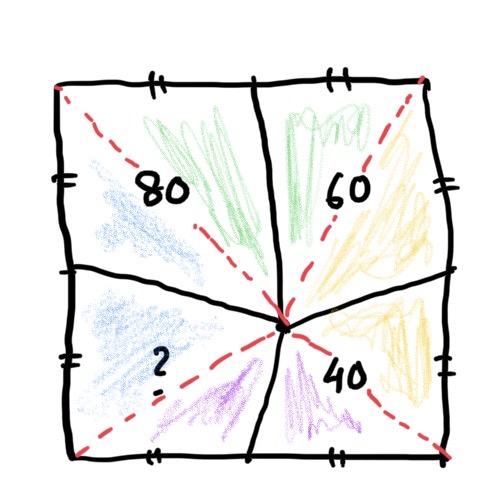6 May 2020

# Answer for the square puzzle

To get to the answer, first let’s visualize the quadrants a little differently. Draw a line (the red dotted ones here in the picture) from the point in the square to each of the vertices. So, the four quadrilaterals have been now divided into eight triangles. Here comes a twist – instead of thinking about the two triangles in any quadrilateral, think about any triangle and the other adjacent one from it. (other than the one in its own quadrilateral).

In the picture, I have colored them. The two greens, the two yellows etc. What can you say about the two green triangles? If you remember old geometry, they have the same base (half a side of the square) and same height. Thus the two green triangles have same areas. Similarly, the two yellows have same area. As do the two blue. And the two purple.

Now think about the quadrilateral with area 80 and that of the one with 40 (diagonally opposite).  One green + one blue + one yellow + one purple. And that is 80+40=120.

Take the other two quadrilaterals. You will notice that they also add up to one green + one blue + one yellow + one purple. Which means the unknown quadrilateral has to be 120 – 60 = 60.

Thus the area of the square is 80+60+40+60=240 units !1

Posted May 6, 2020 by Rajib Roy in category "Puzzles

2 COMMENTS :

1.By Aditi on

What if the quadrilateral of unknown area is opposite 80? Then

green + yellow = 60
yellow + purple = ?
purple + blue = 40
blue + green = 80

So, 60+40 = green + yellow + purple + blue = 80 + ?

then the unknown quadrilateral is 20.
The area of the square is 200.

I have not run additional checks on whether this configuration is actually possible. So maybe I am missing something obvious.

1.By Rajib Roy (Post author) on

Aditi, as you pointed out, if 80 was opposite the unknown area, it would have been 200. The other possible combination is 80 is opposite 60. In which case the square will be 280. Two Opposite quadrilaterals wIll always add up to the other two (and half of the square). With 40,60,80, any combination (in all 3) is possible.

If it were 20, 60 and 80, though, then only two combinations would be possible. You could not have 20 and 60 as opposite quadrilaterals. Then the other quadrilateral would be 0.

This site uses Akismet to reduce spam. Learn how your comment data is processed.